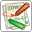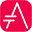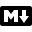# MathsYou can use AsciiMath or JLaTeXMath notation within PlantUML:

@startuml :$int_0^1f(x)dx$; :$x^2+y_1+z_12^34$; note right Try also $d/dxf(x)=lim_(h->0)(f(x+h)-f(x))/h$ <latex>P(y|\mathbf{x}) \mbox{ or } f(\mathbf{x})+\epsilon</latex> end note @endumlor:

@startuml Bob -> Alice : Can you solve: $ax^2+bx+c=0$ Alice --> Bob: $x = (-b+-sqrt(b^2-4ac))/(2a)$ @enduml## Standalone diagramYou can also use @startmath/@endmath to create standalone AsciiMath formula.

@startmath f(t)=(a_0)/2 + sum_(n=1)^ooa_ncos((npit)/L)+sum_(n=1)^oo b_n\ sin((npit)/L) @endmathOr use @startlatex/@endlatex to create standalone JLaTeXMath formula.

@startlatex \sum_{i=0}^{n-1} (a_i + b_i^2) @endlatex## How is this working ?To draw those formulas, PlantUML uses two OpenSource projects:

• AsciiMath that converts AsciiMath notation to LaTeX expression.
• JLatexMath that displays mathematical formulas written in LaTeX. JLaTeXMath is the best Java library to display LaTeX code.

ASCIIMathTeXImg.js is small enough to be integrated into PlantUML standard distribution.

Since JLatexMath is bigger, you have to download it separately, then unzip the 4 jar files (batik-all-1.7.jar, jlatexmath-minimal-1.0.3.jar, jlm_cyrillic.jar and jlm_greek.jar) in the same folder as PlantUML.jar.